Courses

# Soil Mechanics - 2

## 10 Questions MCQ Test GATE Civil Engineering (CE) 2022 Mock Test Series | Soil Mechanics - 2

Description
This mock test of Soil Mechanics - 2 for Civil Engineering (CE) helps you for every Civil Engineering (CE) entrance exam. This contains 10 Multiple Choice Questions for Civil Engineering (CE) Soil Mechanics - 2 (mcq) to study with solutions a complete question bank. The solved questions answers in this Soil Mechanics - 2 quiz give you a good mix of easy questions and tough questions. Civil Engineering (CE) students definitely take this Soil Mechanics - 2 exercise for a better result in the exam. You can find other Soil Mechanics - 2 extra questions, long questions & short questions for Civil Engineering (CE) on EduRev as well by searching above.
QUESTION: 1

### Which one of the following is the most active clay material?

Solution: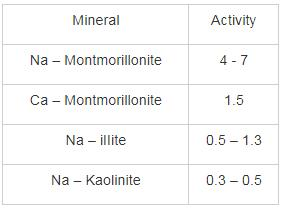QUESTION: 2

### What will be a range of plasticity index for the fine-grained soil below A - line having intermediate compressibility in the plasticity chart.

Solution:

As liquid limit (w2) for lined grained soils having intermediate compressibility is:

35% ≤ WL ≤ 50%

Ip = 0.73 [WL - 20]

For WL = 35%

Ip = 10.95

For WL = 50%

Ip = 21.9

Range 10.95 ≤ Ip ≤ 21.9

QUESTION: 3

### In an Octahedral unit if Fe2+ is present at the center of the geometry, what will be the net charge present over this unit.

Solution:

In Octahedral unit 6 Hydroxyl atoms encloses Fe2+ (as in this case) at the centre of Octahedral Geometry where in each hydroxyl atom is shared in between 3 Octahedral units.Net charge present = − 6 × 1/3 + 2 = 0

QUESTION: 4

Which of the following is correctly matched: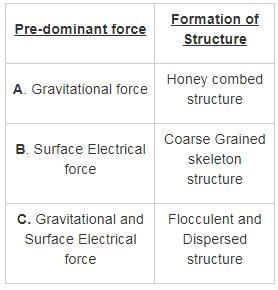Solution:

Coarse Grained skeleton structure: Coarse grained skeleton, is a structure of soil which is present in composite soils containing both fine and coarse-grained particles. But, it is formed when there is large amount of coarse grained particles than the fine-grained particles. The coarse-grained particle forms a skeleton like structure and voids between them are filled by fine grained or clayey particles. it is observed for particles having size greater than 0.02 mm. Gravitational force plays the pre-dominant role in the formation of this structure.

Honey combed structure: Honeycomb structure found in soil contains particle of size 0.02mm to 0.002mm which are generally fine sands or silts. When this type of soils is allowed to settle on the ground, the particles will attract each other and joins one with another and forms a bridge of particles. A large void is also formed between those bridges which makes the soil very loose in nature.it is formed for the soils having particle size is in the range of “0.0002 mm to 0.02 mm”. Both Gravitational and Surface electrical force plays important role in the formation of this structure.

Flocculent and dispersed structure: Flocculated structure is present in clay particles which contains larger surface area. These are charged particles which have positive charge on the edges and negative charge on the face of the particle. When there is net attractive force between the particles, then positive charged particles attracted towards negatively charged faces which results the formation of flocculated structure. It is formed when the size of particle is less than 0.0002 mm. Surface Electrical forces plays the pre-dominant role in the formation of this structure

QUESTION: 5

A soil has a liquid limit of 45% and lies above the A-line when plotted on a plasticity chart. The group symbol of the soil as per IS soil classification is

Solution: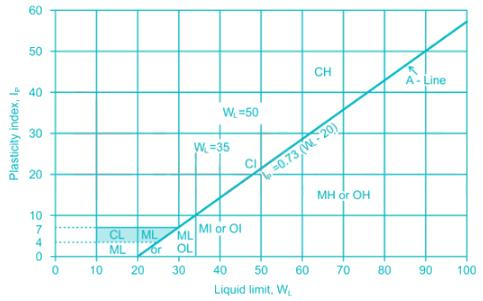QUESTION: 6

Consider the following statements regarding clay particles.

1. The clay particles carry a net negative charge.

2. The cation concentration increases with the distance from the surface of the particle.

3. When the spacing between the two clay particles is very small, the force of attraction is greater than the force of repulsion.

The correct statements are:

Solution:

The clay particles carry a net negative charge on their surfaces. This is the result both of isomorphous substitution and of a break in continuity of the structure at its edges. In dry clay, the negative charge is balanced by exchangeable cations like Ca2+, Mg2+, Na+, and K+ surrounding the particles being held by electrostatic attraction. When water is added to clay, these cations and a few anions float around the clay particles. This configuration is referred to as a diffuse double layer.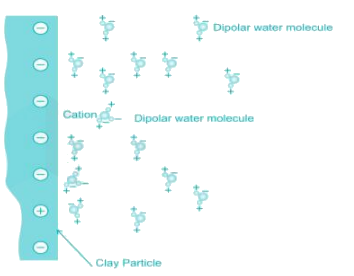The cation concentration decreases with the distance from the surface of the particle. The force of attraction between water and clay decreases with distance from the surface of the particles.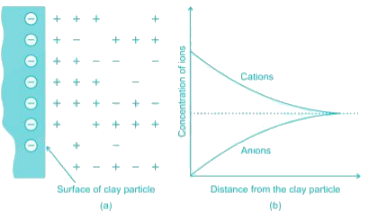When two clay particles in suspension come close to each other, the tendency for interpenetration of the diffuse double layers results in repulsion between the particles. At the same time, an attractive force exists between the clay particles that is caused by van der Waals forces and is independent of the characteristics of water. Both repulsive and attractive forces increase with decreasing distance between the particles, but at different rates. When the spacing between the particles is very small, the force of attraction is greater than the force of repulsion.

QUESTION: 7

The laboratory test results of the soil sample are given below:

Liquid limit = 37%

Plastic limit = 22%

% passing through 75 μ sieve = 26%

% retained over 4.75 mm IS sieve = 65%

% retained over 0.075 mm but passing through 4.75 mm = 26%

As per IS 1498 – 1970, the soil is classified as:

Solution:

% passing through 75 μ sieve = 26%

% retained over 75 μ sieve = 100 – 26% = 74 % > 50%

Hence the soil is coarse grained soil.

As, larger proportion of soil is retained over 4.75 mm IS sieve, so the soil is termed as Gravel.

Now, % fineness = 26% > 12%

So, use Ip to further classify the soil

IP = wL - wP = 37 – 22 = 15%

IP = 15% > 7%

So, soil is termed as clayey Gravel.

QUESTION: 8

A soil mass contains 40 % gravel, 50 % sand 10 %  silt. The soil can be classified as-

Solution: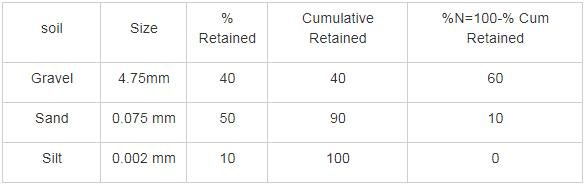D60 = 4.75 mm    and D10 = 0.075mm

Implies             Cu = D60/ D10 = 4.75/0.075 = 63.33

QUESTION: 9

Sieve analysis is conducted on two soils A and B. The results of the test are given below: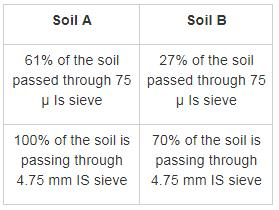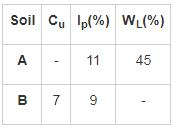Identify the soil on the basis of the result obtained?

Solution:

For Soil A:

More than 50% of the soil fraction passed through 0.075 mm Is sieve is known fine Grained soil

IP(A-line) = 0.73 (WL - 20)

IP = 0.73 (45 - 20) = 18.25

IP for soil = 11

Thus soil lies below A-line of the Plasticity chart. it is known as silt.

WL for soil lies between 35% to 50% WL = 45% (Given)

Soil A is silt of Intermediate Compressibility Soil A = MI

For Soil B:

More than 50% of the soil is retained through 0.075 mm Is sieve. It is termed as coarse Grained soil.

Less than 50% of the soil is retained over 4.75 mm Is sieve, so it is sand

% fineness Given = 27% > 12% and IP = 9% (Given)

i.e. IP > 7%

So, it is termed as SC soil

Soil B = SC

*Answer can only contain numeric values
QUESTION: 10

Sieve analysis is conducted on a given sample of soil. The data collected is given below:

(i) % of particles passing through 75 μ IS sieve = 73%

(ii) Liquid limit of the soil = 50

(iii) Plasticity index of the soil = 16

The possible value of Group index for soil is _______

Solution:

Concept:

Group index of a soil is given by

G.I = 0.2 a + 0.005 ac + 0.01 bd

a = It is the portion of % passing through 75 μ sieve greater than 35 but not exceeding 75 expressed as whole number in between [0-40].

b = It is the portion of % passing through 75 μ sieve greater than 15 but not exceeding 55 expressed as whole number in between [0-40].

c = It is the portion of the numerical liquid limit greater than 40 but not exceeding 60 expressed as whole number in between [0-20].

d = It is the portion of the numerical plasticity index greater than to 10 but not exceeding 30 expressed as whole number in between [0-20].

Calculation:

a = 73 - 35 = 38 < 40

a = 38

b = p - 15

p = 73% which is greater than 55

So, take p = 55

b = 55 - 15 = 40

c = WL - 40

c = 50 - 40 = 10

d = IP - 10

d = 16 - 10 = 6

G.I = 0.2 a + 0.005 ac + 0.01 bd

G.I = 0.2 × 38 + 0.005 × 38 × 10 + 0.01 × 40 × 6

G.I = 11.9